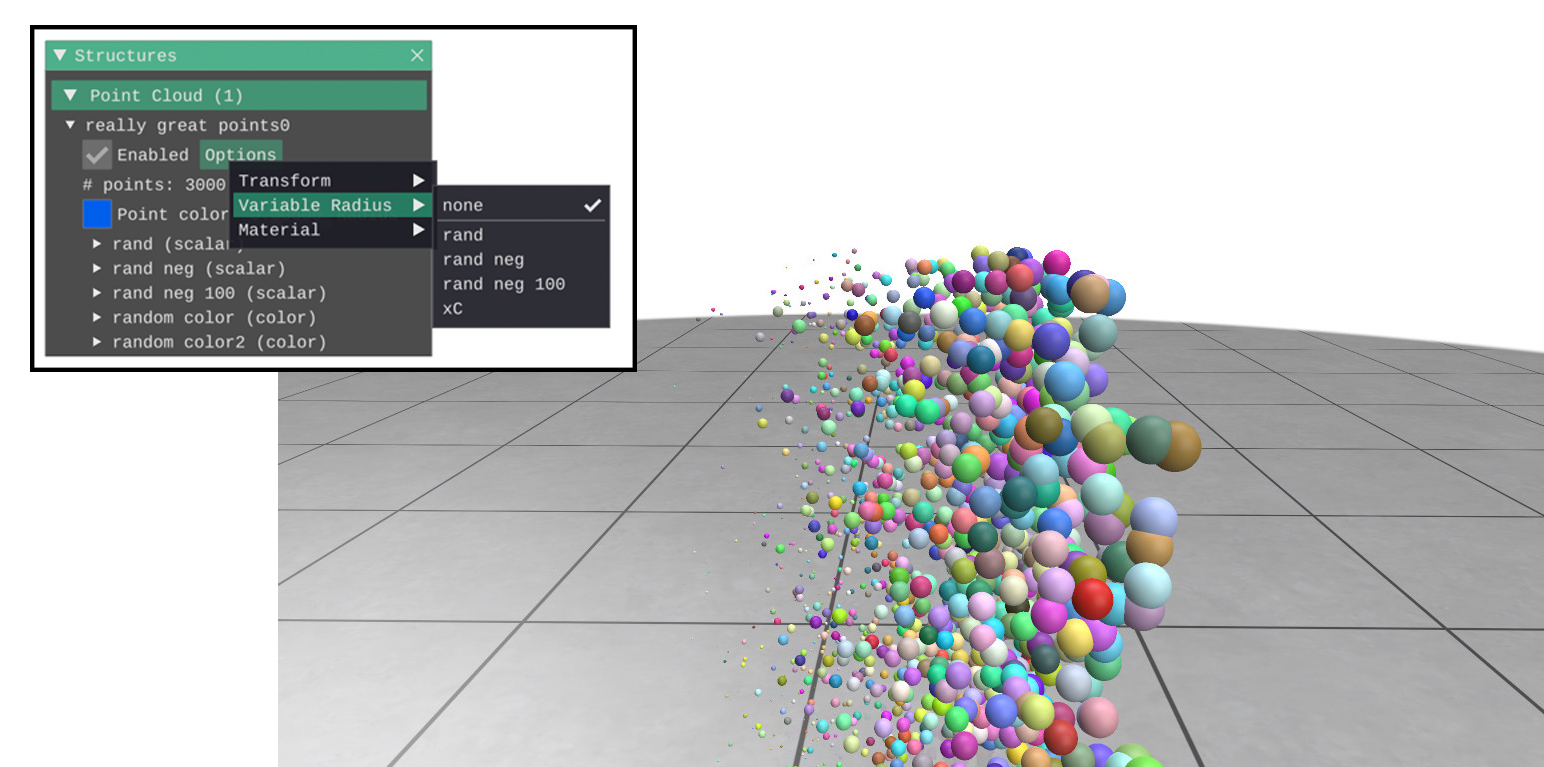By default, all points in the cloud have the same radius. However, any point cloud scalar quantity can be additionally interpreted as the radius of the points. This can also be set manually in the GUI via the point cloud [Options] –> [Variable Radius].

Example:

#include "polyscope/point_cloud.h"

// Populate a random scalar quantity
std::vector<double> xC(points.size());
for (size_t i = 0; i < points.size(); i++) {
xC[i] = points[i].x;
}

// Get a reference to some point cloud
auto psCloud = polyscope::getPointCloud(/* your point cloud name */);

// psCloud->setPointRadiusQuantity("xC"); // equivalently, the name can be usedAny negative values in the scalar quantity will be clamped to 0. By default, values will be rescaled such that the largest corresponds to the size from the point radius option (thus, using any constant scalar quantity will make the radii identical to the default value with no radius set). This automatic scaling can be disabled by setting autoScale=false below.

By default, the point cloud still respects the point radius parameter as adjusted via the slider in the GUI or via setPointRadius(). The variable radius from the quantity is multiplied by the structure parameter to get the actual radius used.

To properly reproduce a radius in world-coordinate units, you can apply the variable quantity without autoscaling like cloud->setPointRadiusQuantity(q, false). This will prevent the auto-scaling of the radii, and also ignore the point radius parameter.

void PointCloud::setPointRadiusQuantity(PointCloudScalarQuantity* quantity, bool autoScale = true)

Set the point radius from a quantity.

When using a radius which is a physical length in world coordinates, set autoScale to false, to remapping and ignore the structure’s point radius parameter.

void PointCloud::setPointRadiusQuantity(std::string name, bool autoScale = true)

Set the point radius from a quantity by name. The quantity must be a point cloud scalar quantity add to this cloud.

When using a radius which is a physical length in world coordinates, set autoScale to false, to remapping and ignore the structure’s point radius parameter.

void PointCloud::clearPointRadiusQuantity()